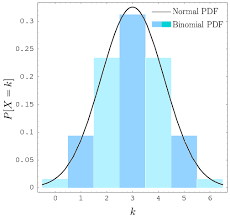## How to Calculate and Solve for General Binomial Distribution | ProbabilityThe image above represents general binomial distribution.

To compute for general binomial distribution, four essential parameters are needed and these parameters are n, r, q and q.

The formula for calculating general binomial distribution:

P(r successes) = n!(n – r)!r!q(n – r)pr

Where;

P(r successes) = General Binomial Distribution
p = P(A)
q = P(not A)

Let’s solve an example;
Find the general binomial distribution when n is 8, r is 6, p is 1 and q is 0.

This implies that;

n = 8
r = 6
p = 1
q = 0

P(r successes) = n!(n – r)!r!q(n – r)pr
P(6 successes) = 8!(8 – 6)!6!(0)(8 – 6)(1)6
P(6 successes) = 8!2!6!(0)2(1)6
P(6 successes) = 40320(2)(720)(0)2(1)6
P(6 successes) = 403201440(0)2(1)6
P(6 successes) = (28)(0)2(1)6
P(6 successes) = (28)(0)(1)
P(6 successes) = 0

Therefore, the general binomial distribution is 0.